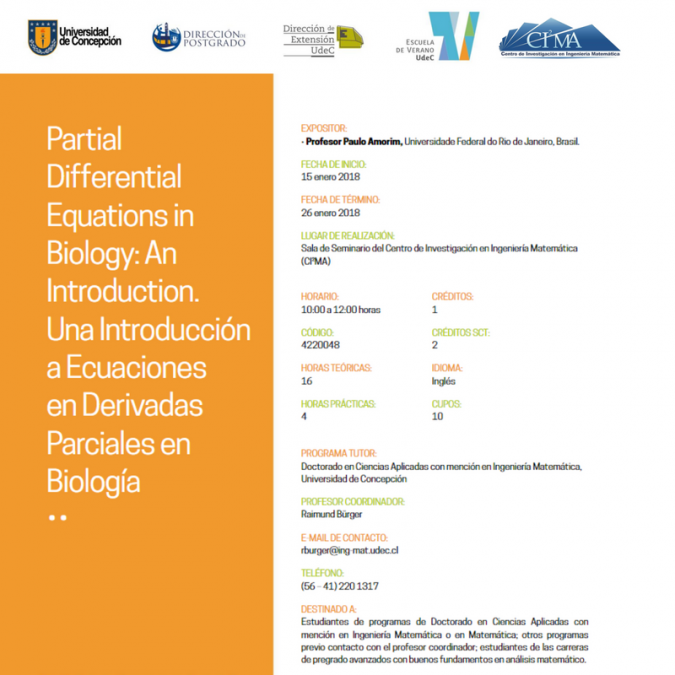﻿﻿ CI²MA - Events | Events of CI²MA

# Events of CI²MA

15 January 2018: Partial Differential Equations in Biology: an introductionDescription: Advanced mathematical models for biological phenomena that evolve in space and time, such as infectious diseases, dynamics of populations, ecological systems, invasions, predator-prey systems, food chains,  movement of bacteria, chemotaxis, and pattern formation, can be formulated in terms of a partial differential equation or a system of such equations. Such a mathematical model  relates the rate of change of a quantity (typically, the density of a species or the concentration of a substance) at a given position with the value and spatial gradients of the same and other unknowns.  Typical ingredients include spatially heterogeneous environments, the variability of climate due to marked different seasons, birth and death processes, and effects of human inteference such as harvesting, vaccination, and  travel activity. It is the purpose of this course to provide an introduction to the mathematical theory of selected partial differential equations that arise in this context, including creating   familiarity with the types of PDE appearing in biology and the underlying modeling strategies; the analysis of parabolic systems of equations arising in this context; the study of weak solutions; an exploration of the phenomenon of finite time blow-up and chemotaxis; hyperbolic equations; and a simple kinetic equation.

Speaker: Profesor Paulo Amorim, Universidade Federal do Rio de Janeiro, Brazil. Reserch visit funded by Graduate School from UdeC and CMM-Basal Project

First session: 01/15/2018

Final sesion: 01/26/2018

Location:  Meeting Room at Center for Research in Mathematical Engineering (CI²MA)

Time: 10:00 AM to noon